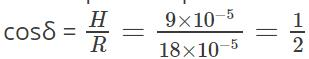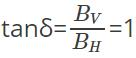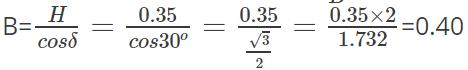Courses

# Test: Earth's Magnetism

## 10 Questions MCQ Test Physics For JEE | Test: Earth's Magnetism

Description
This mock test of Test: Earth's Magnetism for JEE helps you for every JEE entrance exam. This contains 10 Multiple Choice Questions for JEE Test: Earth's Magnetism (mcq) to study with solutions a complete question bank. The solved questions answers in this Test: Earth's Magnetism quiz give you a good mix of easy questions and tough questions. JEE students definitely take this Test: Earth's Magnetism exercise for a better result in the exam. You can find other Test: Earth's Magnetism extra questions, long questions & short questions for JEE on EduRev as well by searching above.
QUESTION: 1

### How many quantities are required to specify the magnetic field of the earth?

Solution:

Three quantities are needed to specify the magnetic field of the earth on its surface, namely, the horizontal component, the magnetic declination and the magnetic dip. These are known as elements of the earth’s magnetic field or magnetic elements.

QUESTION: 2

### Which of the following is the definition for magnetic meridian of Earth?

Solution:

A vertical plane passing through the axis of a freely suspended or pivoted magnet is known as magnetic meridian of Earth. The vertical plane passing through the geographical North Pole and South Pole at a given place is known as the geographical meridian of that place.

QUESTION: 3

### Which among the following is denoted by δ?

Solution:

Magnetic dip or inclination at a place is defined as the angle made by the Earth’s magnetic field with the horizontal in the magnetic meridian. It is denoted by δ.
Magnetic declination at a place is defined as the angle between the geographic meridian and magnetic meridian.

QUESTION: 4

The Earth always have both horizontal and vertical components everywhere.

Solution:

No, this statement is false. The Earth always has a vertical component except at the equator. Similarly, the earth always has a horizontal component except at the poles.

QUESTION: 5

Identify the expression for horizontal component from the following.

Solution:

Horizontal component is a component of Earth’s magnetic field along the horizontal direction in the magnetic meridian. It is denoted by BH. If B is the intensity of Earth’s total magnetic field, then the horizontal component of Earth’s magnetic field is given by:
BH=Bcosδ

QUESTION: 6

At the magnetic North Pole of the Earth, what is the value of the angle of dip?

Solution:

Angle of dip is 90o at geographical North Pole because attraction on the North Pole of needle is very strong and the needle remains in vertical plane. At the magnetic equator, the needle will point horizontally, i.e. dip angle is 0o. As you move from the magnetic equator towards the magnetic pole, the angle increases in the northern hemisphere. So, the angle of dip is maximum.

QUESTION: 7

At a given place on the Earth’s surface, the horizontal component of Earth’s magnetic field is 9 × 10-5 T and the resultant magnetic field is 180 × 10-6. Calculate the angle of dip at this place.

Solution:

Given: H = 9 × 10-5; R = 180 × 10-6 = 18 × 10-5
The required equation ➔ H=RcosδTherefore, δ = cos-1 1/2

=60o
Thus, the angle of dip is 60o.

QUESTION: 8

When is the angle of dip at a place equal to 45o?

Solution:

Yes, the angle of dip at a place is equal to 45o, when the vertical and horizontal components of earth’s magnetic field are equal. In this case ➔ δ=45oSo, BV=BH.

QUESTION: 9

The angle of dip at a certain place on Earth is 30o and the magnitude of Earth’s horizontal component of magnetic field is 0.35 G. Find the magnetic field at that place on Earth.

Solution:

Given: Horizontal component (H) = 0.35 G; Angle of dip (δ) = 30o
Required equation ➔ cosδ= H/BTherefore, the magnitude of the magnetic field at that place is 0.40 G.

QUESTION: 10

What is the relation between angle of dip and magnetic latitude?

Solution:

If a magnet is freely suspended or inclined or dipped, the angle between the horizontal and the angle of inclination of the magnet that is inclined only by the earth’s magnetic field will indicate the magnetic latitude. The relation is as follows:
tanδ=2tanλ